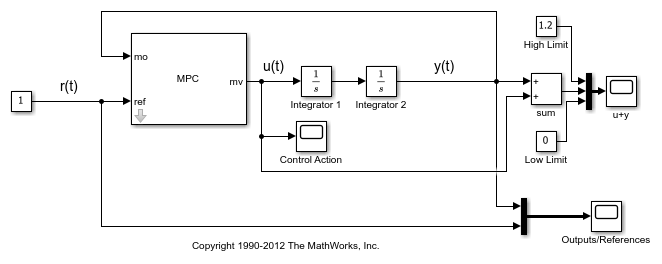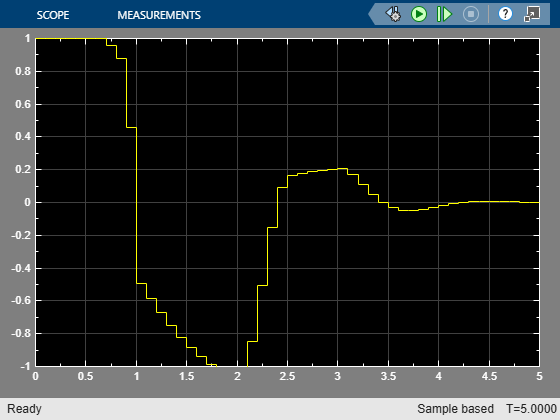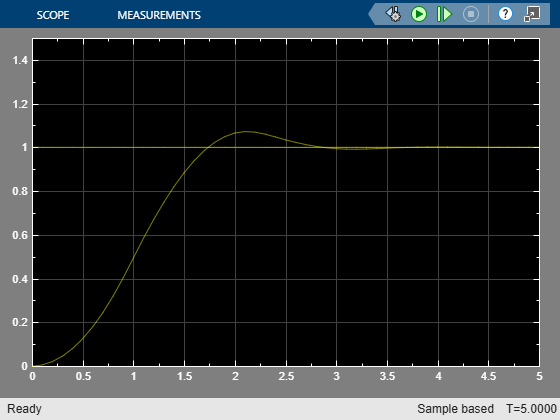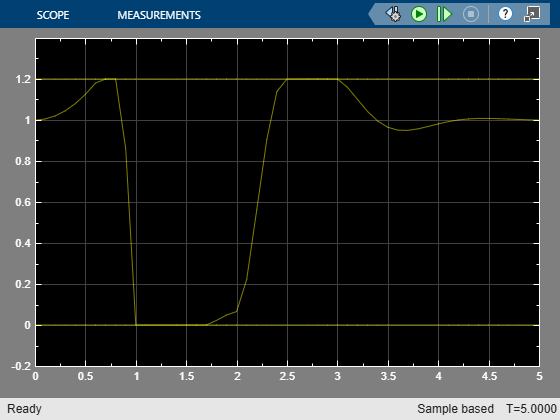Documentation

### This is machine translation

Mouseover text to see original. Click the button below to return to the English version of the page.

## Using Custom Input and Output Constraints

This example shows how to design model predictive controller with mixed input/output constraints.

### Design MPC Controller

The basic setup of the MPC controller includes:

• A double integrator as the prediction model

• Prediction horizon of 20

• Control horizon of 20

• Input constraints `-1 <= u(t) <= 1`

```plant = tf(1,[1 0 0]); % Prediction model Ts = .1; % Sampling time p = 20; % Prediction horizon m = 20; % Control horizon mpcobj = mpc(plant,Ts,p,m); % MPC object mpcobj.MV = struct('Min',-1,'Max',1); % Input saturation constraints ```
```-->The "Weights.ManipulatedVariables" property of "mpc" object is empty. Assuming default 0.00000. -->The "Weights.ManipulatedVariablesRate" property of "mpc" object is empty. Assuming default 0.10000. -->The "Weights.OutputVariables" property of "mpc" object is empty. Assuming default 1.00000. ```

### Define Mixed Input/Output (I/O) Constraint

The sum of the input `u(t)` and output `y(t)` must be nonnegative and smaller than 1.2:

` 0 <= u(t) + y(t) <= 1.2`

To impose this combined (mixed) I/O constraint, formulate it as a set of inequality constraints involving `u(t)` and `y(t)`:

``` u(t) + y(t) <= 1.2 -u(t) + -y(t) <= 0```
```setconstraint(mpcobj,[1;-1],[1;-1],[1.2;0]); ```

To run this example, Simulink® is required.

```if ~mpcchecktoolboxinstalled('simulink') disp('Simulink(R) is required to run this example.') return end ```

Simulate closed-loop control of the linear plant model in Simulink. Controller "mpcobj" is specified in the block dialog.

```mdl = 'mpc_mixedconstraints'; open_system(mdl); % Open Simulink(R) Model sim(mdl); % Start Simulation ```
```-->Converting the "Model.Plant" property of "mpc" object to state-space. -->Converting model to discrete time. Assuming no disturbance added to measured output channel #1. -->The "Model.Noise" property of the "mpc" object is empty. Assuming white noise on each measured output channel. ```As you can see, the MPC Controller always keeps the sum `u+y` between 0 and 1.2 while tracking the reference signal `r=1`.

```bdclose(mdl); ```